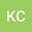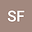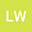Least energy sign-changing solutions for a class of fractional $(p,q)$-Laplacian problems with critical growth in $\R^N$
•••ShengHao Feng
Nanchang University
Author Profileli wang
East China Jiao Tong University
Author Profile## Abstract

In this paper we consider the following fractional $(p,q)$-Laplacian equation $$(-\Delta)_{p}^{s} u+(-\Delta)_{q}^{s} u+V(x)\left(|u|^{p-2} u+|u|^{q-2} u\right)=\lambda f(u)+|u|^{q^*_s-2}u \quad \text { in } \mathbb{R}^{N},$$ where \$s \in(0,1), \lambda>0, 2